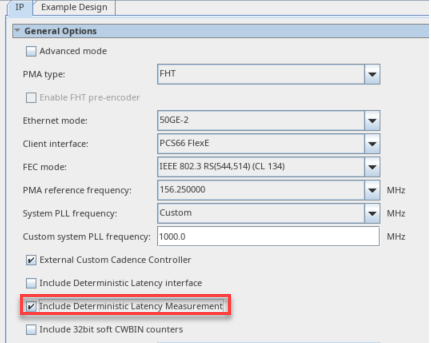ID 683023
Date 4/03/2023
Public

## 7.12.1. Deterministic Latency Measurement

Deterministic Latency (DL) is the ability to precisely determine the delay between the FPGA core and the serial pins. In most applications, the variability is acceptable to determine the actual delay within a given reset session. This section provides an example that shows the calculation delay between pins and FPGA core for the F-Tile Ethernet Intel® FPGA Hard IP core.

The Deterministic Latency Interface is an option in which the signals required for latency calculation are exposed to the top of the IP and you need to write RTL logic to measure it.

The Deterministic Latency Measurement is an option in which the F-Tile Ethernet Intel® FPGA Hard IP performs the measurement calculations and store the values in soft registers. You can obtain the latency values by directly accessing the soft CSR registers.

The deterministic latency measurement methodology for Intel® Agilex™ 7 F-tile devices derives from measuring the time when a given word is present at the interface to the PMA, and when that same word arrives at the FPGA core. The difference in time between these two events, when added to the PMA propagation delay, determines the total latency between the FPGA core and the serial pins. Such a calculation intrinsically includes all delays due to intermediate logic, FIFOs, and all other effects.
Figure 54. Enable Deterministic Latency Measurement IP Parameter EditorWhen the deterministic latency measurement is enabled, you can calculate the latency using the deterministic latency factors listed below.

Table 57.  Deterministic Latency Factors
Factor Description
TxDL Transmitter delay in i_sampling_clock cycle.

To calculate the TxDL value, read the DL soft register 0x314 bit[20:0]. The register provides the value in fixed point format. Bit[20:8] represents an integer, and bit[7:0] represents a fractional number.

For example:
• Bit[20:8] = 0x27, the integer value is 39.
• Bit[7:0] = 0xF4, the fractional value is 0.953125.
Therefore, the total delay is 39.953125 clock cycles.
Note: These values are available in simulation output.
RxDL Receiver delay in i_sampling_clock cycle.

To calculate the RxDL value, read the DL soft register 0x318 bit [20:0]. The register provides a value in fixed point format. Bit[20:8] represents an integer, and bit[7:0] represents a fractional number.

For example:
• Bit[20:8] = 0x27, the integer value is 39.
• Bit[7:0] = 0xF4, the fractional value is 0.953125.
Therefore, the total delay is 39.953125 clock cycles.
Note: These values are available in simulation output.
i_sampling_clock_period For F-Tile Ethernet Intel FPGA Hard IP core:
• i_sampling_clk is 250 MHz.
• Period is 4 ns.
eth_wa Obtained from the Datapath Avalon Memory-Mapped Interface register 0x1110[6:0].
ethlphy_wa RS-FEC codeword bit position on Rx value (lowest 5 bit) obtained from the Datapath Avalon Memory-Mapped Interface register 0x6174[4:0].
dlpulse Obtained from the Datapath Avalon Memory-Mapped Interface register 0x1110.
Table 58.  Deterministic Latency EquationsThe table below depicts the deterministic latency equations of TX and RX data paths for different variants.
Latency (ns) For 25GE/50GE/100GE/200GE/400GE with RS-FEC Variants For 10GE/25GE/50GE/100GE without RS-FEC variants
TX TxDL * 4ns + 6 * o_clk_pll_period + TX_PMA_Delay * UI_value
RX RxDL * 4ns - 6 * o_clk_pll_period + (RX_PMA_Delay - ethlphy_wa) * UI_value RxDL * 4ns - 6 * o_clk_pll_period + (RX_PMA_Delay - eth_wa - 33 * dlpulse)* UI_value